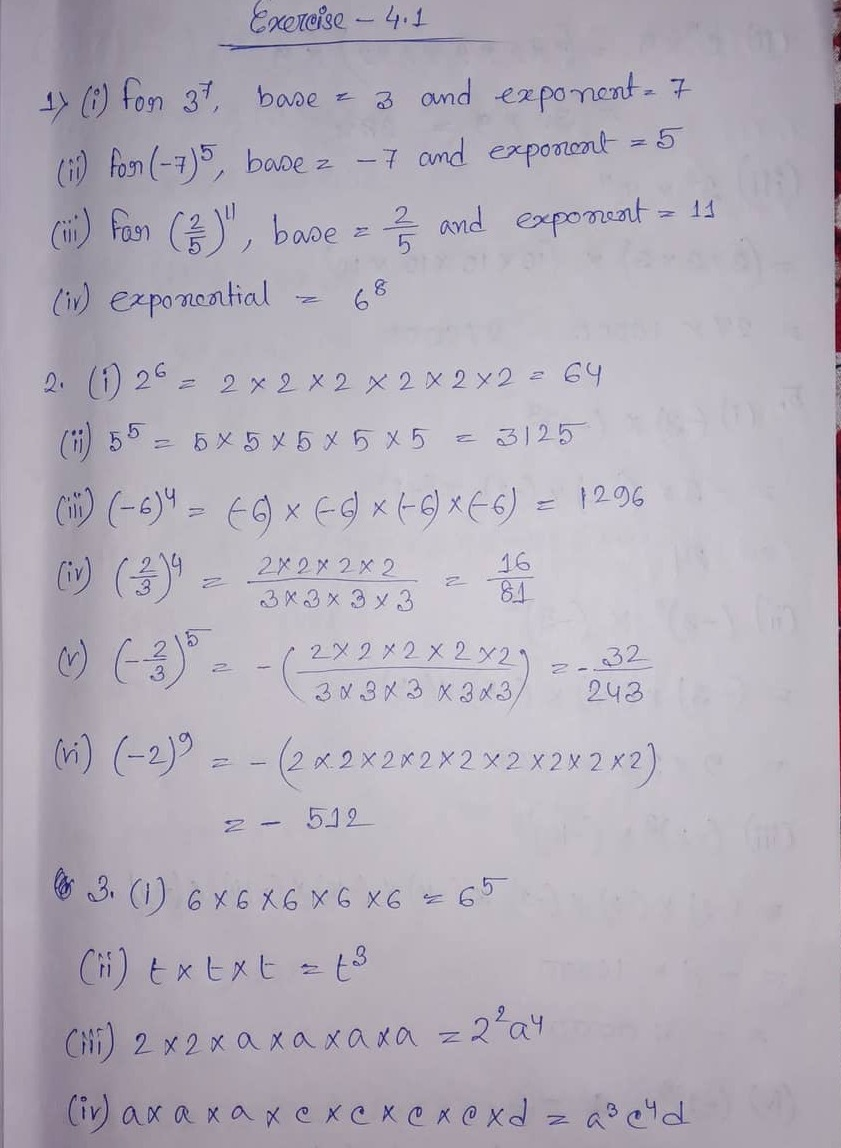# ML Aggarwal CBSE Solutions Class 6 Math Fourth Chapter Exponents and Powers Exercise 4.1

### ML Aggarwal CBSE Solutions Class 6 Math 4th Chapter Exponents and Powers Exercise 4.1

Ex 4.1:

(1) Fill in the blanks:

(i) In the expression 37, base = …. And exponent = …..

(ii) In the expression (-7)5, base = ….. and exponent = ……

(iii) In the expression (2/5)11 base = …. And exponent = …..

(iv) If base is 6 and exponent is 8, then exponential form = ____

(2) Find the value of the following:

(i) 26

(iii) 55

(iii) (-6)4

(iv) (2/3)4

(v) (-2/5)5

(vi) (-2)9

(3) Express the following in the exponential form:

(i) 6 x 6 x 6 x 6 x 6

(ii) t x t x t

(iii) 2 x 2 x a x a x a x a

(iv) a x a x a x c x c x c x c x d

Solution:(4) Simplify the following

(i) 7 x 103

(ii) 25 x 9

(iii) 33 x 104

(5) Simplify the following

(i) (-3) x (-2)3

(ii) (-3)2 x (-5)2

(iii) (-2)3 x (-10)4

(iv) (-1)9

(v) 252 x (-1)31

(vi) 42 x 33 x (-1)122

(6) Identify the greater number in each of the following:

(i) 43 or 34

(ii) 73 or 37

(iii) 45 or 54

(iv) 210 or 102

Solution:

(i) 7 x 103

= 7 x (10 x 10 x 10)

= 7 x 1000

= 7000(7) Write the following numbers as power of 2:

(i) 8

(ii) 128

(iii) 1024

(8) To what power (-2) should be raised to get 16?

Solution:(9) Write the following numbers as powers of (-3):

(i) 9

(ii) -27

(iii) 81

(10) Find the value of x in each of the following:

(i) 7x = 343

(ii) 3x = 729

(iii) (-8)x = -512

(iv) (-4)x = -1024

(v) (2/5)x = 32/3125

(vi) (-3/4)x = – 243/1024

(11) Write the prime factorization of the following numbers in the exponential form:

(i) 72

(ii) 360

(iii) 405

(iv) 540

(v) 2280

(vi) 3600

(vii) 4725

(viii) 8400

Solution:Updated: December 16, 2019 — 12:19 pm